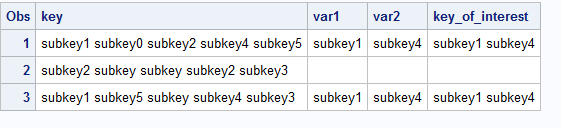## how to write a variable that maps to several variable in a data step

Hello team,

I have a variable called key and this variable is mapped to many subkeys. Both variables are present in the table.

1-key variable has many values

2- Each key maps to a couple of subkeys.

3-How can I write the program in a data step that I only capture a couple of subkeys for each key, I don't need all of them.

4-Do I need to put it in if else statement? If yes, how can I reach there?

Thanks,

blue

Blue Blue
5 REPLIES 5

## Re: how to write a variable that maps to several variable in a data step

Examples of your data would go a long way towards clarifying what you actually want to do.

If the dataset has variables named KEY and SUBKEY with multiple values of SUBKEY per value of KEY and is already sorted by KEY SUBKEY you can make a subset pretty easily using a simple data step.

Just count how many subkeys you have seen and delete the extra observations.

``````data want;
set have;
by key subkey;
if first.key then subkey_number = 0;
if first.subkey;
subkey_num + 1;
if subkey_num > 2 then delete;
keep key subkey subkey_num;
run;``````

## Re: how to write a variable that maps to several variable in a data step

One example;

``````data have;
infile cards dlm=',' truncover;
length key \$100;
input key \$;
cards;
subkey1 subkey0 subkey2 subkey4 subkey5,
subkey2 subkey subkey subkey2 subkey3,
subkey1 subkey5 subkey subkey4 subkey3
;
proc print;run;

data want;
set have;
if find(key, 'subkey1', 'i') gt 0 then var1='subkey1';
if find(key, 'subkey4', 'i') gt 0 then var2= 'subkey4';
key_of_interest= catx(' ', of var:);
proc print; run; ``````## Re: how to write a variable that maps to several variable in a data step

Why is subkey1 and subkey4 chosen here? Is that something that can be hard-coded, or is the problem much more general than that? If so, explain in detail.

--
Paige Miller

## Re: how to write a variable that maps to several variable in a data step

As per description by the owner (@GN0001) :

2- Each key maps to a couple of subkeys.

3-How can I write the program in a data step that I only capture a couple of subkeys for each key, I don't need all of them.

Assuming the user interested in mapping only 'subkey1' and 'subkey4' among all 'subkey' s, those values were mapped to a new Key variables. But it could be any 'subkey', not only those 2.

## Re: how to write a variable that maps to several variable in a data step

@A_Kh wrote:

As per description by the owner (@GN0001) :

2- Each key maps to a couple of subkeys.

3-How can I write the program in a data step that I only capture a couple of subkeys for each key, I don't need all of them.

Assuming the user interested in mapping only 'subkey1' and 'subkey4' among all 'subkey' s, those values were mapped to a new Key variables. But it could be any 'subkey', not only those 2.

Thanks @A_Kh there's a good chance you are right, but we need to hear that from @GN0001 along with a lot more detail.

--
Paige Miller
Discussion stats
• 5 replies
• 264 views
• 1 like
• 4 in conversation